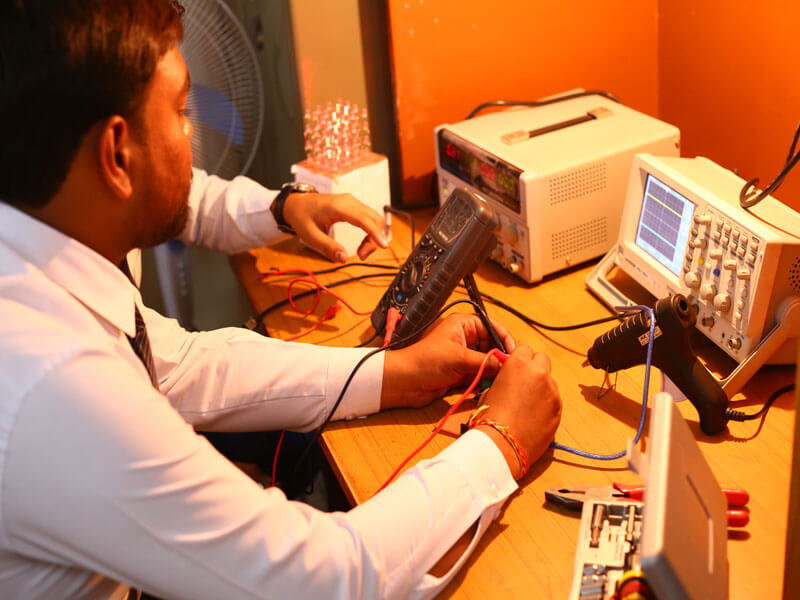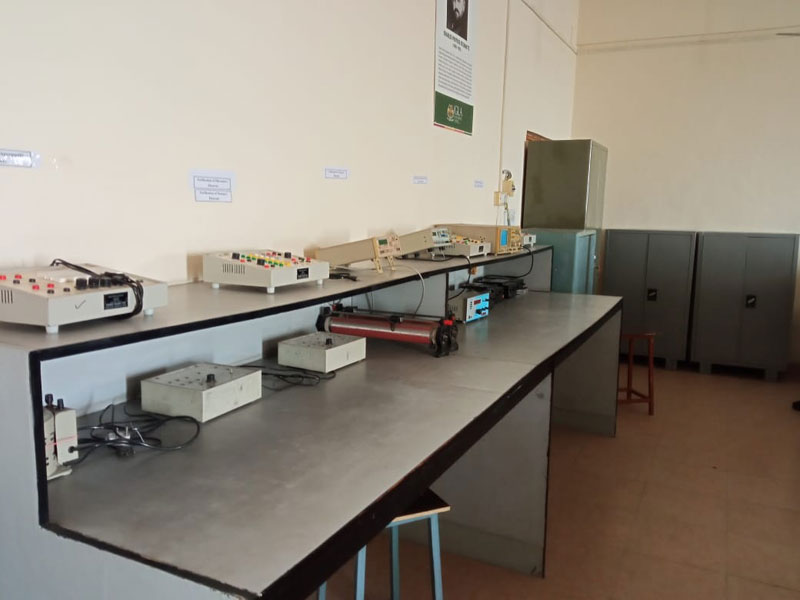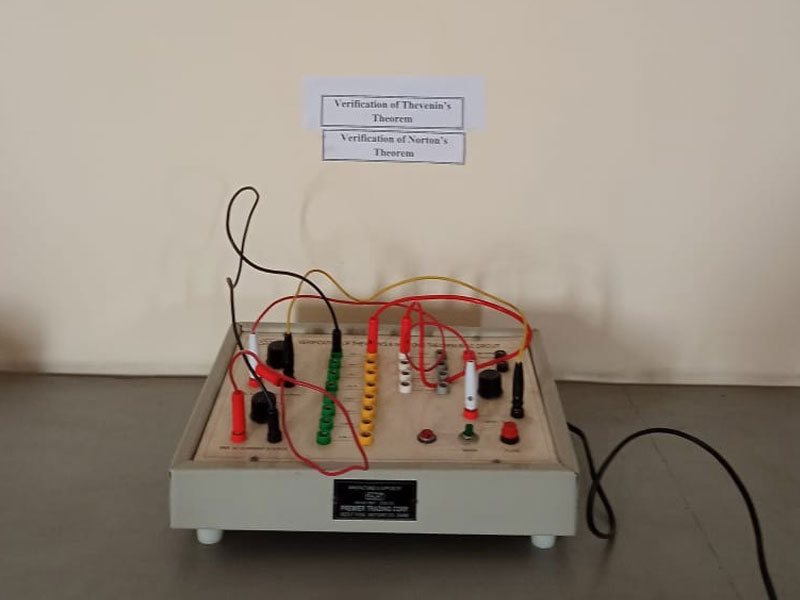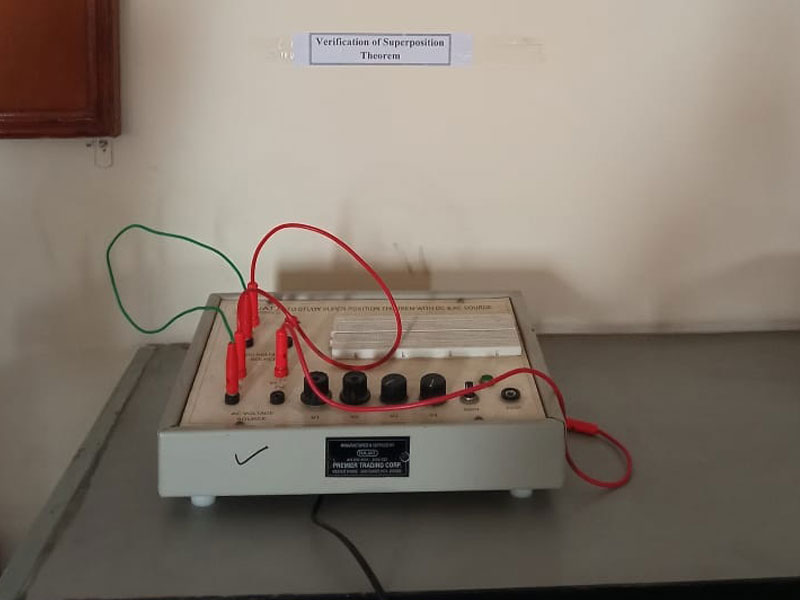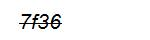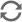### Network Lab

Electrical Network theory is one of the most important course in electrical engineering which is used for understanding the basic circuit laws and theorems, analysis of circuit using different methods and synthesis of networks. In network lab, students are able to verify different laws and theorems practically. They also do the same on PSpice software. The main objective of this lab is for the training on basic network topologies, verification of various laws and theorems for both AC and DC circuits.

Major Equipments:

• Digital Multimeter
• Digital Storage Oscilloscope
• Function Generator
• Autotransformer
• Analogue terminal panel for laboratory work and experimentation
• Verification of Thevenin’s and Norton’s theorem, Superposition Theorem for AC circuit kit
• Determination of Two – port parameters

Software Available: